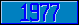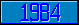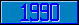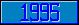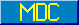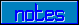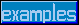## \$%COTH^MATH

### M[UMPS] by Example

Approved for inclusion in a future M[UMPS] language standard as part of the library for Hyperbolic trigonometric Mathematics.

This function returns the hyperbolic cotangent of the value of its parameter (in radians).

Reference Value \$%COTH^MATH(–5) –1.000090804... \$%COTH^MATH(–2) –1.037314721... \$%COTH^MATH(–1) –1.313035285... \$%COTH^MATH(0) "INFINITE" \$%COTH^MATH(1) 1.313035285... \$%COTH^MATH(2) 1.037314721... \$%COTH^MATH(5) 1.000090804...

The MDC has approved code to approximate the return value of this function. Implementors are encouraged to provide more accurate and efficient code).